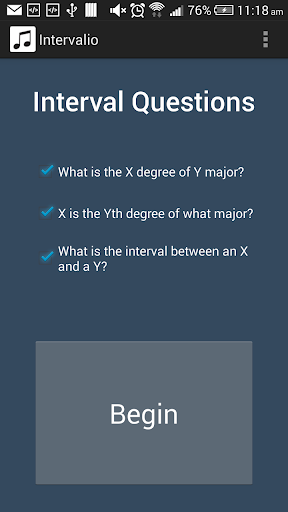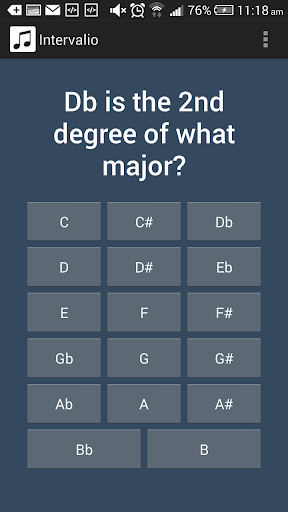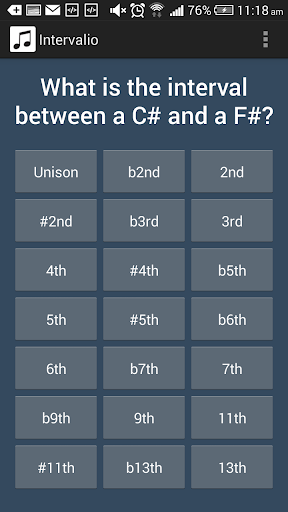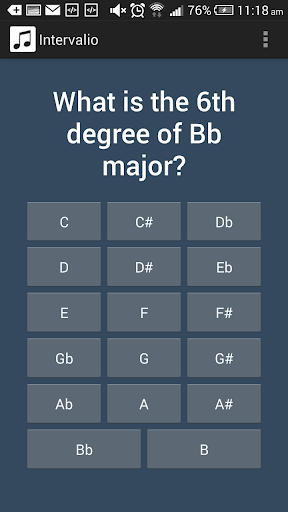# IntervalioChoose from a combination of three questions:

What is the X degree of Y major?

X is the Yth degree of what major?and What is the interval between an X and a Y?

Press all correct answers to proceed to the next question.

Note: "What is the interval between an X and a Y" questions go both ways; find all intervals starting at X going to Y, and find all intervals starting at Y going to X. We also use enharmonics, so D# is the same as Eb, and #4th is the same as b5th. Also remember that 2nd degree is the same as the 9th, 4th is the same as the 11th, and the 6th is the same as the 13th.